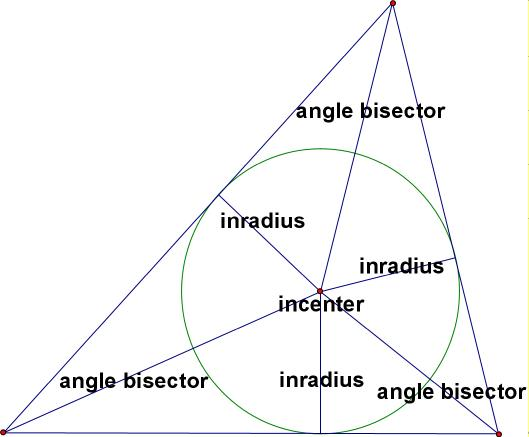index: click on a letter A B C D E F G H I J K L M N O P Q R S T U V W X Y Z A to Z index index: subject areas numbers & symbols sets, logic, proofs geometry algebra trigonometry advanced algebra & pre-calculus calculus advanced topics probability & statistics real world applications multimedia entrieswww.mathwords.com about mathwords website feedback

 Inscribed Circle Incircle The largest possible circle that can be drawn interior to a plane figure. For a polygon, a circle is not actually inscribed unless each side of the polygon is tangent to the circle. Note: All triangles have inscribed circles, and so do all regular polygons. Most other polygons do not have inscribed circles. For a regular polygon, the inradius (the radius of the inscribed circle) is called the apothem. Inscribed Circle of a TriangleSee also